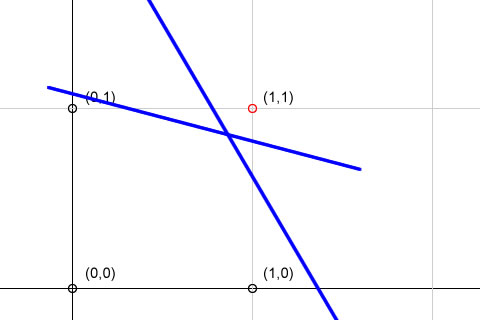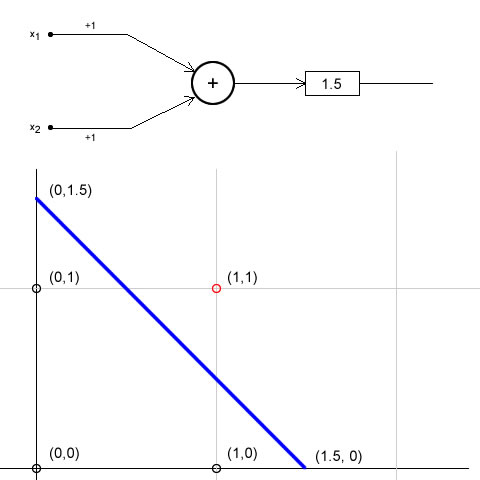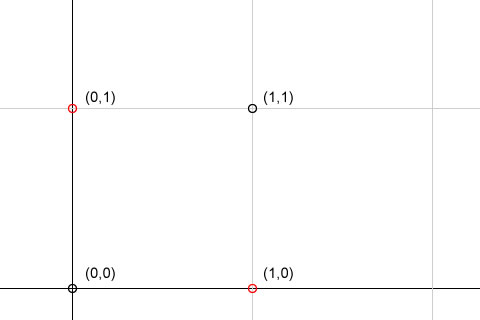## Dictomizer examples: AND/OR gates

Now that you know how a dictomizer works, we'll work on some more examples. I'll leave the mathematical calculates for you. Make sure you do them!

### AND gate

We'll create a 2 input AND gate.

Since there are two inputs, we can assume this to be a 2 dimensional Cartesian plane. Something like this:The black points belong to one class (for them the output is 0). And the red point belongs to another class (the output is 1).

So we know the inputs and the output.

All thats left to find is the weights and the threshold value T. Then, we'd have a hardwired neuron to do the AND operation.

We notice that one single line is sufficient to do this classification. There exist infinitely many lines that do separate the black points from the red one. But any ONE will be sufficient for us.

So, only one single neuron is needed to do this "classification". And the weights of inputs for that single neuron will be equal to the coefficients of the separating line.

I leave this up to you. Take any random line that separates the black and the red points. Then find it's equation. Then you'll have the weights (the coefficients of x and y) and the thershold value T (the constant in the line's equation). Here's a sample neuron hardwired to do this task:### OR gate

We'll try creating a 3-input OR gate. Now, in this case, we'll have to consider a 3-D Cartesian space (because we have 3 inputs).

Now I want you to imagine a cube. Each edge is 1 unit in length. So, the various vertices of the cube will be (0,0,0) or a (1,0,1), or a (1,1,1). So, all possible inputs will be covered if we assume a 3D cube.

Next I want you to imagine the OR operation at the vertices. Only the (0,0,0) input will give an output of 0 (o OR o OR o = 0). Any other vertex will have atleast one input that is non zero.

So, only (0,0,0) belongs to one class. All other vertices belong to another class. Now try imagining a plane that separated (0,0,0) from the rest of the vertices.

The coefficients of x, y, and z in this plane's equation will be the weights of the 3 inputs. And the constant will be the threshold T. Again, there exist infinitely many planes that do the job. But only one is sufficient. So we need a single neuron.

You should be able to workout on your own from here :)

### The XOR gate

Dictomizers are not the answer to all your problems. Here's an example that simply is NOT possible with a single neuron dictomizer.You simply cannot find one single line that separates the red points from the black points. You need at least two lines. This means you need two neurons. We'll get to this example at a later stage. When we've done multi-neuron neural networks.

#### Related posts#### 7 unique neural network architectures